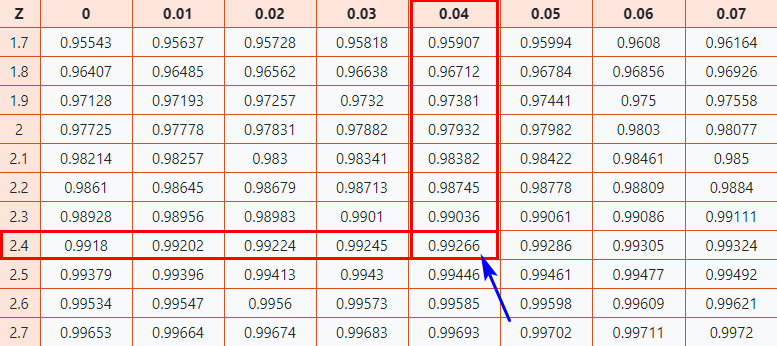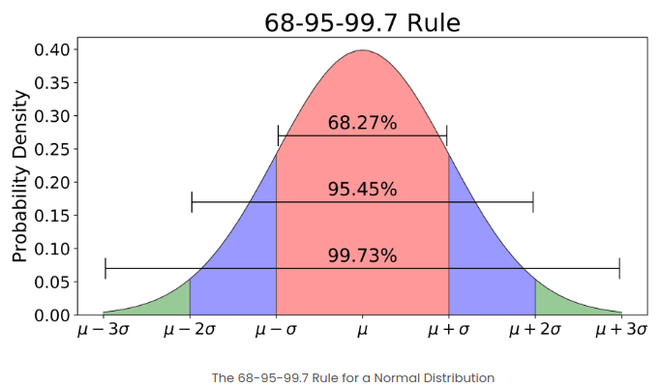# Z-Score in Statistics

• Difficulty Level : Easy
• Last Updated : 27 May, 2021

In this article, we are going to discuss the following concepts,

• What’s a Z-Score?
• Formula for Z-Score
• How to Calculate Z-Score?
• Interpretation of Z-score

### What’s a Z-Score?

Z-score is also known as standard score gives us an idea of how far a data point is from the mean. It indicates how many standard deviations an element is from the mean. Hence, Z-Score is measured in terms of standard deviation from the mean. For example, a standard deviation of 2 indicates the value is 2 standard deviations away from the mean. In order to use a z-score, we need to know the population mean (μ) and also the population standard deviation (σ).

### The Formula for Z-Score

A z-score can be calculated using the following formula.

#### z = (X – μ) / σ

where,
z = Z-Score,
X = The value of the element,
μ = The population mean, and
σ = The population standard deviation

### How to Calculate Z-Score?

Usually, the population mean ((μ), the population standard deviation (σ), and the observed value (x) are provided in the problem statement, and substituting the same in the above Z-score equation yields us the Z-Score value. Depending upon whether the given Z-Score is positive or negative, one makes use of the respective positive Z-Table or negative Z-Table available online or on the back of your statistics textbook in the appendix.

Example:

Question:
You take the GATE examination and score 500. The mean score for the GATE is 390 and the standard deviation is 45. How well did you score on the test compared to the average test taker?

Solution:
The following data is readily available in the above question statement
Raw score/observed value = X = 500
Mean score = μ = 390
Standard deviation = σ = 45

By applying the formula of z-score,

z = (X – μ) / σ
z = (500 – 390) / 45
z = 110 / 45 = 2.44

This means that your z-score is 2.44

Since the Z-Score is positive 2.44, we will make use of the positive Z-Table.

Now let’s take a look at Z Table (CC-BY) to know how well you scored compared to the other test-takers.

Follow the instruction below to find the probability from the table.

Here,  z-score = 2.44

1. Firstly, map the first two digits 2.4 on the Y-axis.
2. Then along the X-axis, map 0.04
3. Join both axes. The intersection of the two will provide you the Z-Score value you’re looking forAs a result, you will get the final value which is 0.99266.

Now, we need to compare how our original score of 500 on the GATE examination compares to the average score of the batch. To do that we need to convert the Z-Score into a percentage value.

0.99266 * 100 = 99.266%

Finally, you can say that you have performed well than almost 99% of other test-takers.

### Interpretation of Z-score

• An element having a z-score less than 0 represents that the element is less than the mean.
• An element having a z-score greater than 0 represents that the element is greater than the mean.
• An element having a z-score equal to 0 represents that the element is equal to the mean.
• An element having a z-score equal to 1 represents that the element is 1 standard deviation greater than the mean; a z-score equal to 2, 2 standard deviations greater than the mean, and so on.
• An element having a z-score equal to -1 represents that the element is 1 standard deviation less than the mean; a z-score equal to -2, 2 standard deviations less than the mean, and so on.
• If the number of elements in a given set is large, then about 68% of the elements have a z-score between -1 and 1; about 95% have a z-score between -2 and 2; about 99% have a z-score between -3 and 3. This is known as the Empirical Rule or the 68-95-99.7 Rule and can be demonstrated in the image belowMy Personal Notes arrow_drop_up